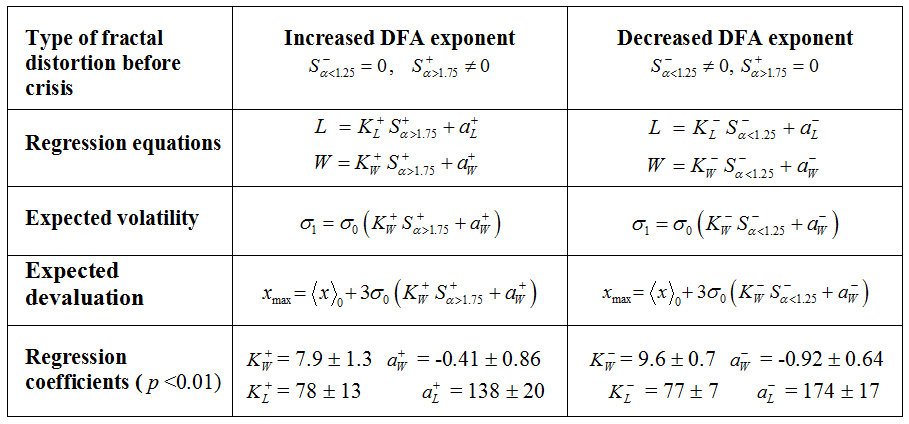# New Methods in Crisis Modeling (Proc. FISS MASR, 2005)

Results of fractal analysis of daily exchange rate fluctuations of floating currencies for a 10-year period are presented. It is shown that monetary crashes are usually preceded by prolonged periods of abnormal fractal exponent. Regression relations between duration and magnitude of currency crises and the degree of distortion of monofractal patterns of exchange rate dynamics are found and have been used as a basic ingredient of a forecasting technique which provided correct in-sample predictions of monetary crisis magnitude and duration over various time scales.

O. Y. Uritskaya. Fractal Methods for Modeling and Forecasting of Currency Crises // Modelling and Analysis of Safety and Risk in Complex Systems. – Proc. FISS MASR, SPb., 2005. – pр. 210-215.( Fourth International Scientific School MASR – 2005 (Saint-Petersburg, Russia, June 28- July 1, 2005)

Full text (in Russian)Fig. 1 . Fractal regression model of currency crises. Using the results of the analysis of correlations between monetary crashes and fractal dynamics of exchange rate fluctuations, we have constructed a regression model allowing quantitative prediction of important crisis parameters (duration L, normalized magnitude W, maximum amount of currency devaluation x_max, exchange rate volatility sigma_1) using statistical characteristics of currency dynamics before the crisis (cumulative deviation S of the DFA exponent below 1.25 and above 1.75 levels, mean value x_0 and standard deviation sigma_0 of currency fluctuations). Basic model equations as well as the values of empirically obtained regression coefficients are shown in the table.  To estimate the performance of the constructed model, statistics of the predicted and values expressed as a percentage of the actual values observed during most significant currency crises which occurred during the studied period has been investigated. On average, the error of x_max in-sample prediction was only 5 %. The prediction of L was characterized by a larger discrepancy(~10%) which may reflect the fact that the crisis length is affected by fiscal measures undertaken by national governments during the period following its beginning stage.